# Introduction to Trigonometry

Fundamental Definitions:

Unit Circle: A circle with the center at the origin and radius of length 1 unit is called unit circle or trigonometric circle on the plane.

The origin of a unit circle is O(0,0)

A(1,0),  B(0,1),  C(-1,0),  D(0,-1)

Let P(x,y) be any point on the unit circle.

In the ;

Directed Angles: An angle is the union of two rays whose initial point is common.

In the figure =[OA U [OB

The angle is formed by rotating a ray [OA  about its end point in a fixed plane.

is positive angle.

is negative angle.

Examples: Graph directed angles 20o, -110o, 360o, 180o, -180o. Show angles on unit circle.

Measures of Angles: An angle is determined by its direction and magnitude and can be either (+) or (-) according to its direction.

360 Degrees: The measurement of the central angle corresponding to the complete arc of a circle is called “360 Degrees” Degree is denoted by the symbol (o). As a result the measurement of a round angle is 360o.

of 1o is called 1’ minute

of 1’ is called 1’’ second

1’=()o

1’’=()’=()o

Example:  and  are given. Find and

400 Grad: The measurement of the central angle corresponding to the complete arc of a circle is called “400 Grads”. Hence, the round angle in grad is called 400G.

When we divide the complete arc of a circle into 400 equal arcs, we obtain 400 equal central angles corresponding to these arcs. The measurement of the central angle corresponding to any one of these arcs is said to be 1G.

2p Radians: The measurement of the central angle corresponding to the complete arc of a circle is called “2p Radians”. Hence, the round angle in radian is 2p.

When the arc of a circle has the same length as the radius of the circle, the measure of its central angle is said to be 1 radian.

If the length of  is L and radius of circle is r then the measure of the central angle is

Example: If L=6, r=2 then find =?

Solution:

Degree-Radian-Grad Conversion Formula: For the complete arc of a circle we see that:

The Angle in Standard Position and Coterminal Angles: The angle whose vertex is at the origin and whose initial ray is the positive x-axis is said to be “in standard position”. The angles whose terminal sides coincide with each other when they are in standard position are said to be coterminal.

Primary Directed Angles: We can write;

mod(360)

mod(360)

mod(360)

.

.

.

mod(360)

is primary directed angle.

### Просмотр содержимого документа«Introduction to Trigonometry»

Subject: Introduction to Trigonometry Date:Taldykorgan KTL

Duration: 80 Minutes

Classes: 10th Classes

Aim: To teach basic concept of trigonometry

Fundamental Definitions:

Unit Circle: A circle with the center at the origin and radius of length 1 unit is called unit circle or trigonometric circle on the plane.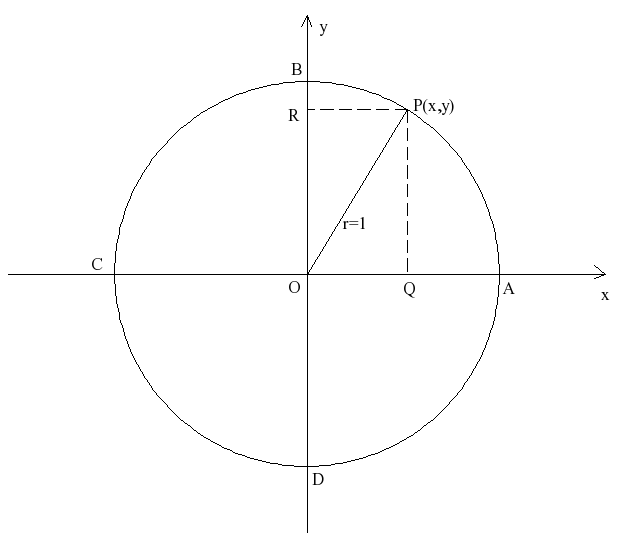The origin of a unit circle is O(0,0) A(1,0), B(0,1), C(-1,0), D(0,-1) Let P(x,y) be any point on the unit circle. In the;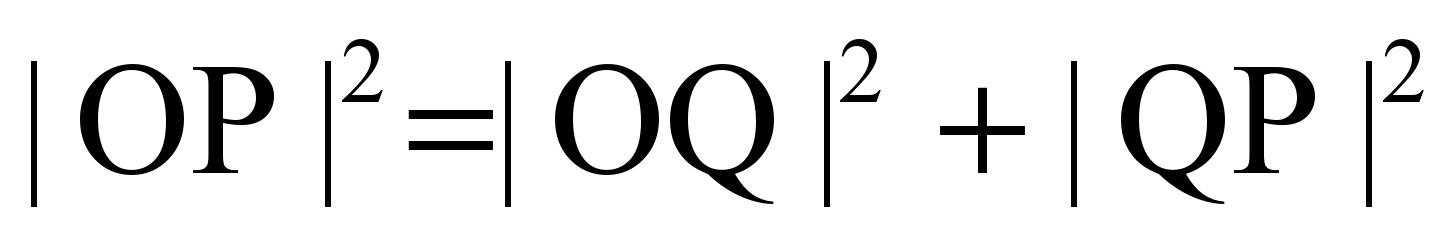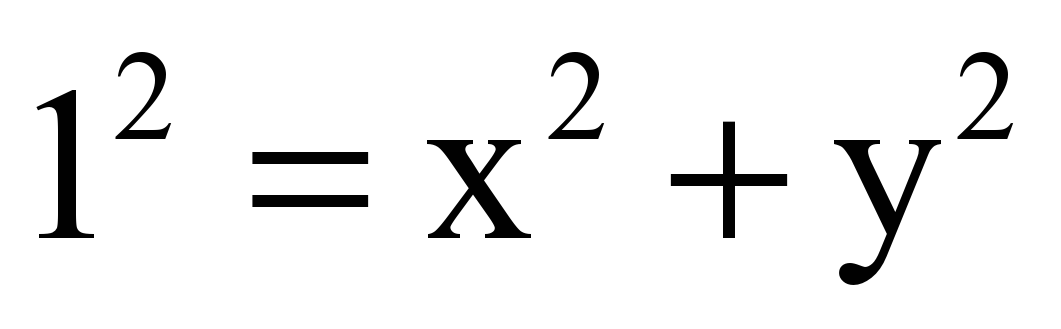Directed Angles: An angle is the union of two rays whose initial point is common.

 In the figure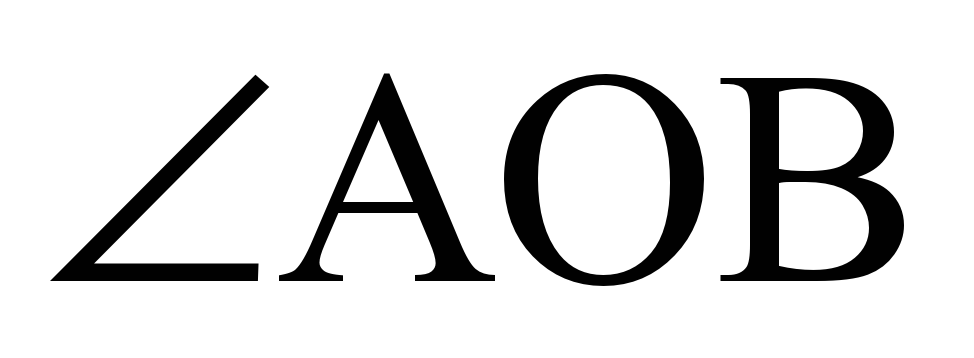=[OA U [OB The angleis formed by rotating a ray [OA about its end point in a fixed plane.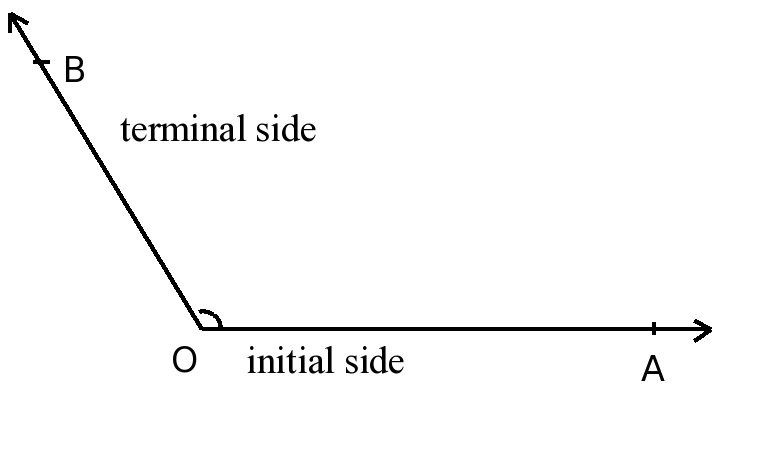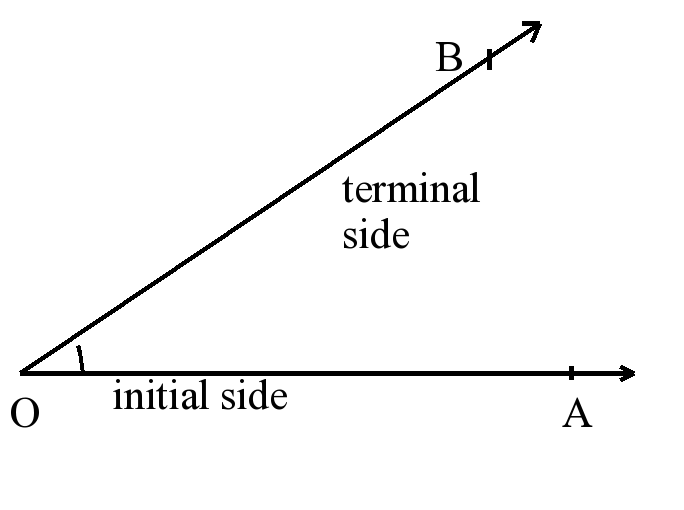is positive angle.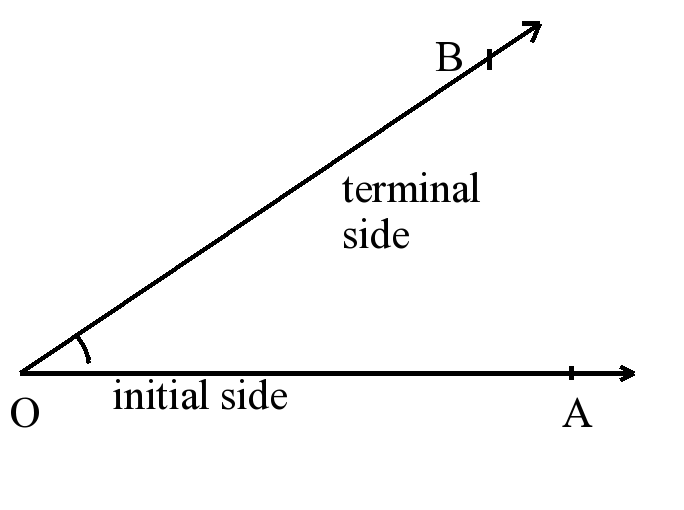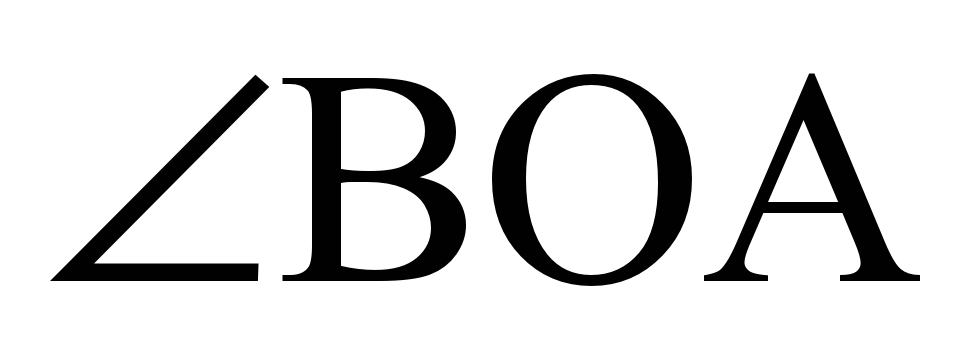is negative angle.

Examples: Graph directed angles 20o, -110o, 360o, 180o, -180o . Show angles on unit circle.

Measures of Angles: An angle is determined by its direction and magnitude and can be either (+) or (-) according to its direction.

360 Degrees: The measurement of the central angle corresponding to the complete arc of a circle is called “360 Degrees” Degree is denoted by the symbol (o). As a result the measurement of a round angle is 360o.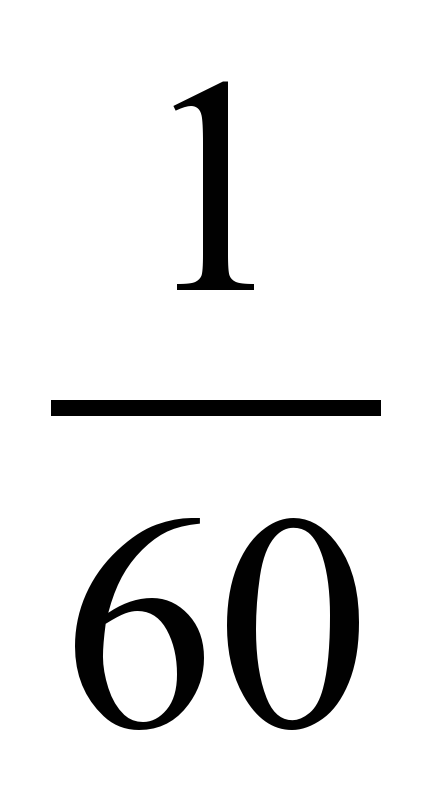of 1o is called 1’ minuteof 1’ is called 1’’ second 1’=()o 1’’=()’=(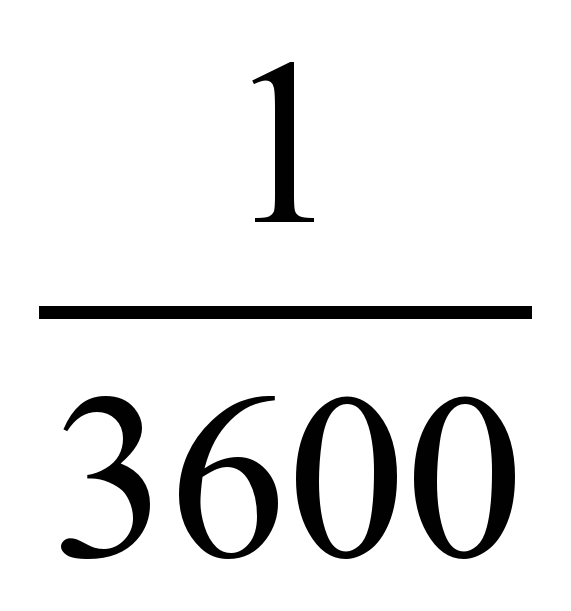)o

Example: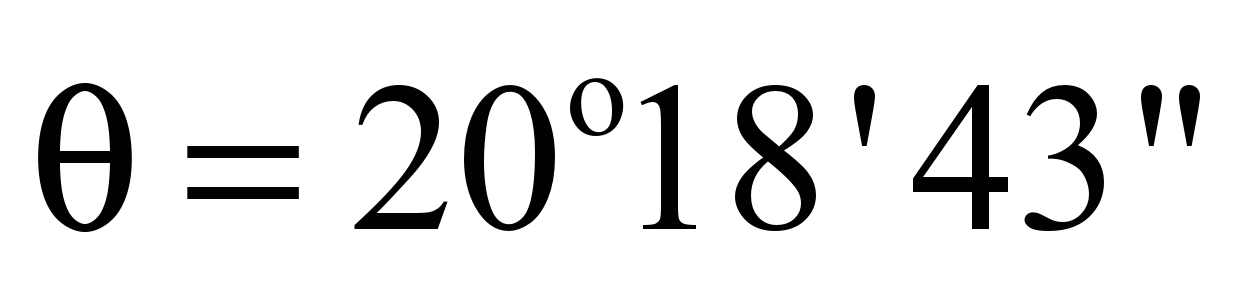and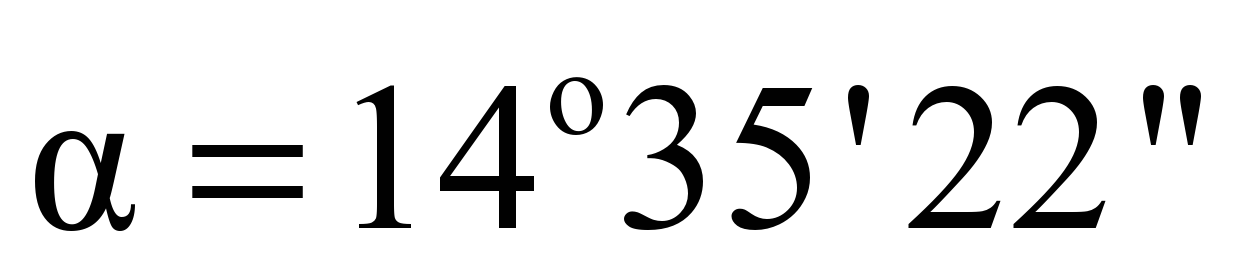are given. Find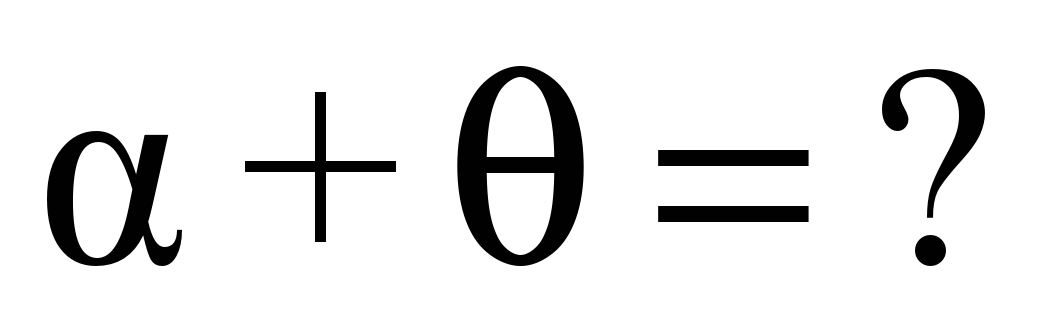and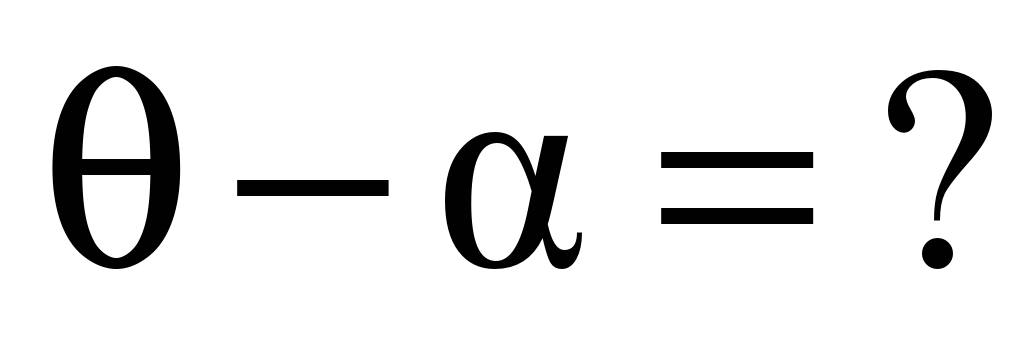400 Grad: The measurement of the central angle corresponding to the complete arc of a circle is called “400 Grads”. Hence, the round angle in grad is called 400G.

 When we divide the complete arc of a circle into 400 equal arcs, we obtain 400 equal central angles corresponding to these arcs. The measurement of the central angle corresponding to any one of these arcs is said to be 1G.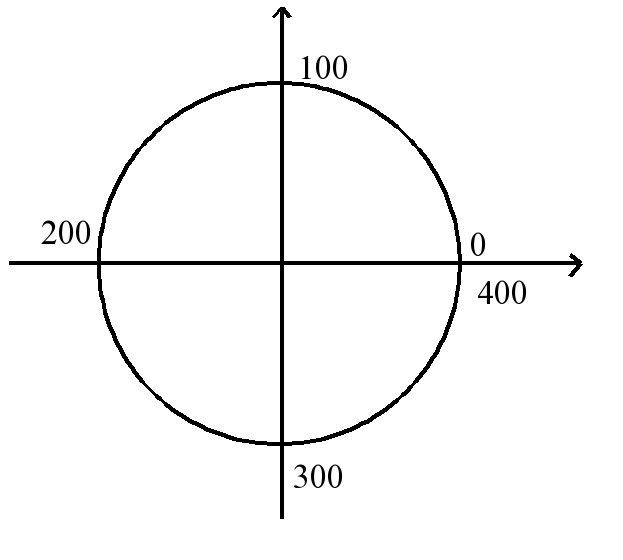2 Radians: The measurement of the central angle corresponding to the complete arc of a circle is called “2 Radians”. Hence, the round angle in radian is 2.

 When the arc of a circle has the same length as the radius of the circle, the measure of its central angle is said to be 1 radian. If the length of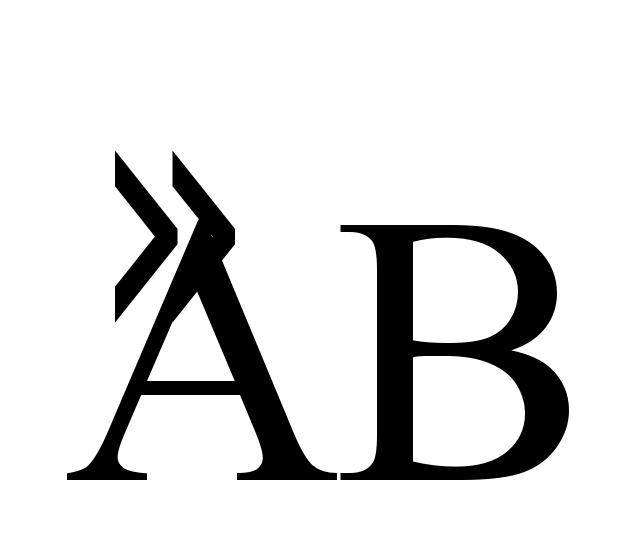is L and radius of circle is r then the measure of the central angle is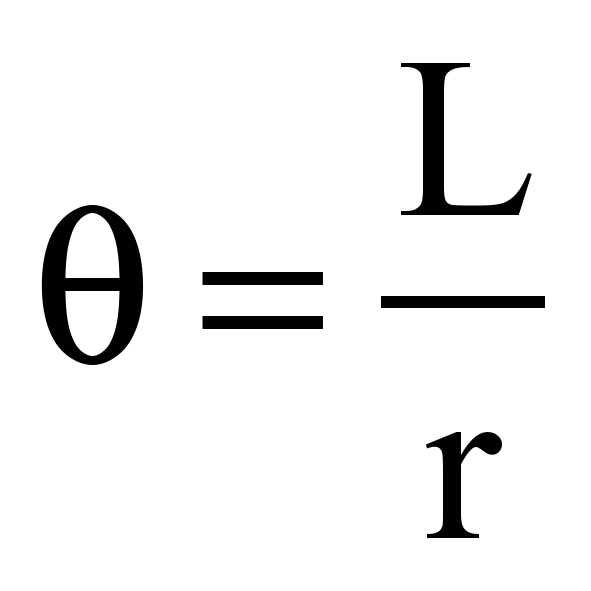in radian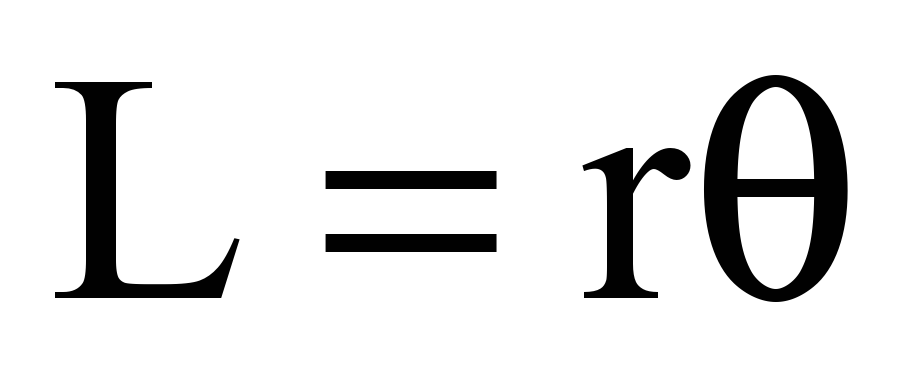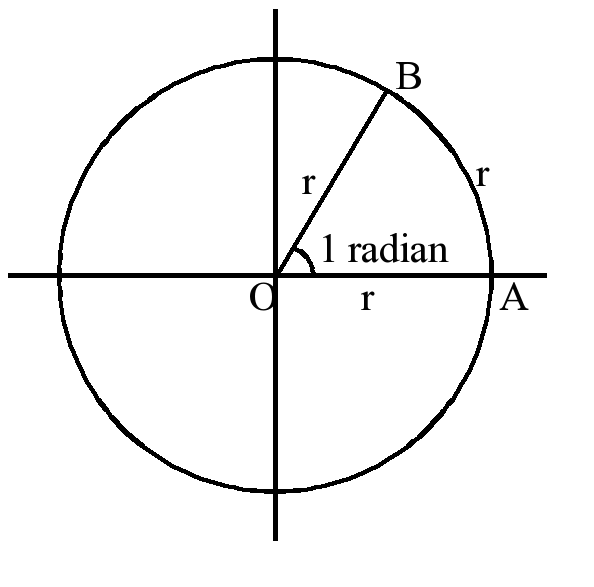Example: If L=6, r=2 then find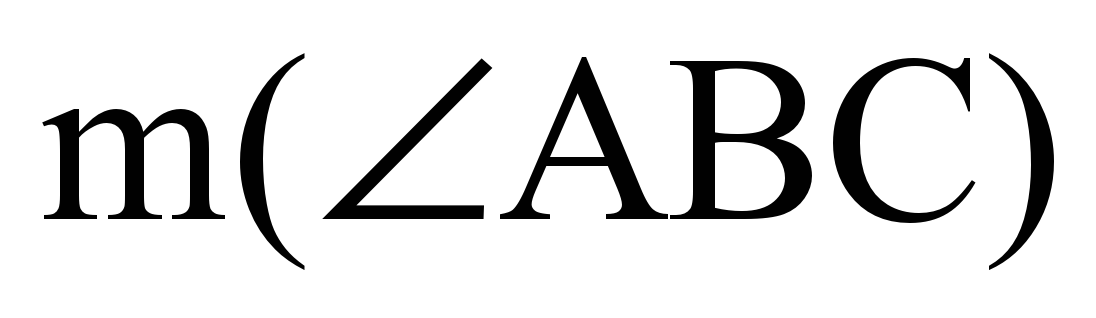=?

Solution: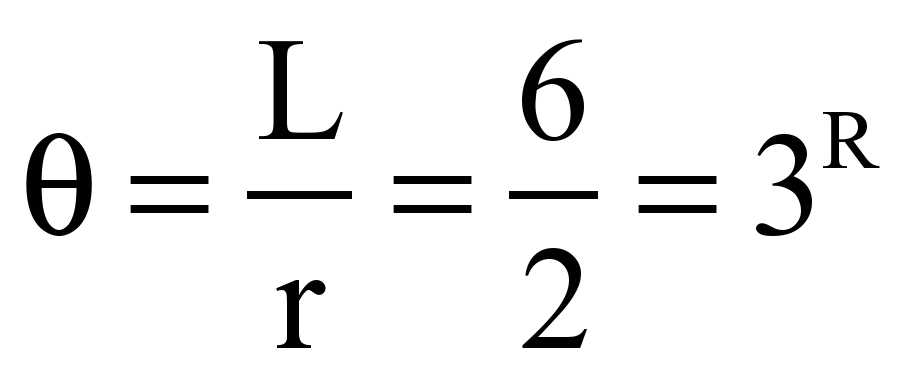Degree-Radian-Grad Conversion Formula: For the complete arc of a circle we see that:

360degrees=2 Radians=400 Grads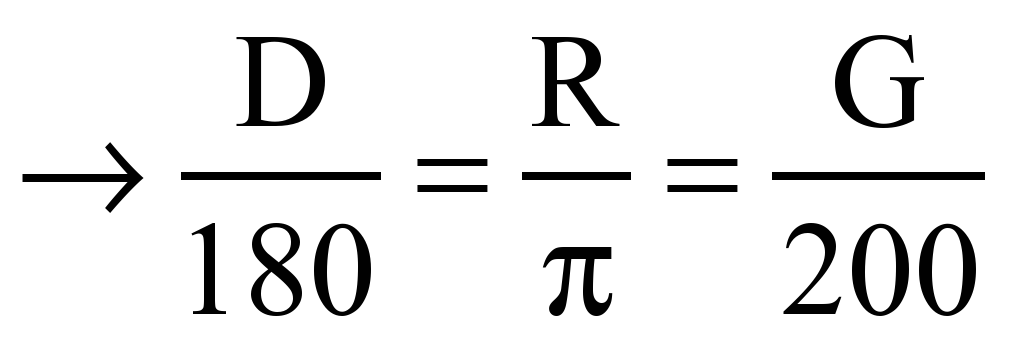Example: Express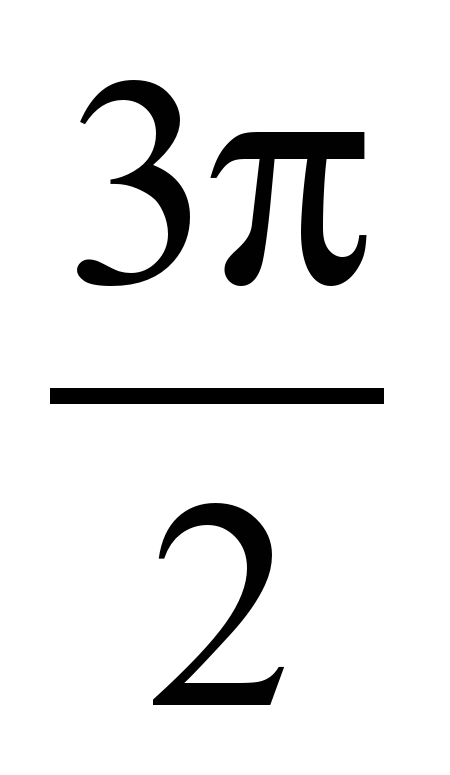radians to degrees.

The Angle in Standard Position and Coterminal Angles: The angle whose vertex is at the origin and whose initial ray is the positive x-axis is said to be “in standard position”. The angles whose terminal sides coincide with each other when they are in standard position are said to be coterminal.

Primary Directed Angles: We can write;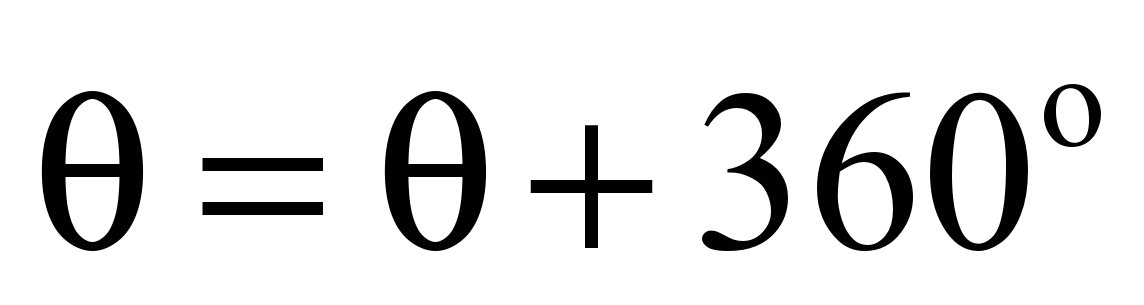mod(360)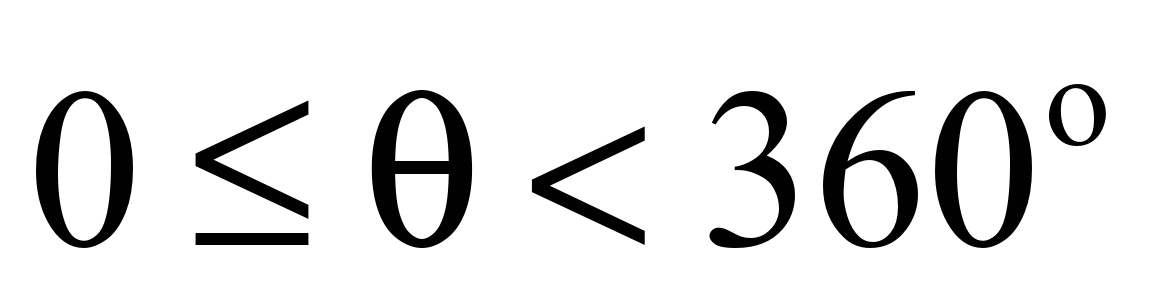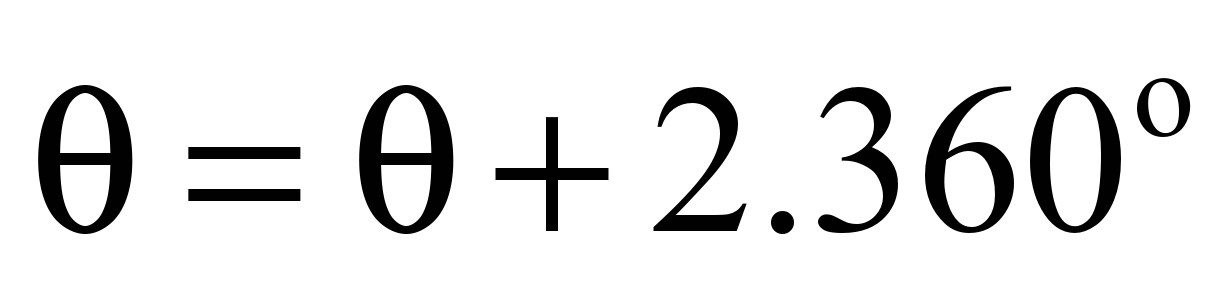mod(360)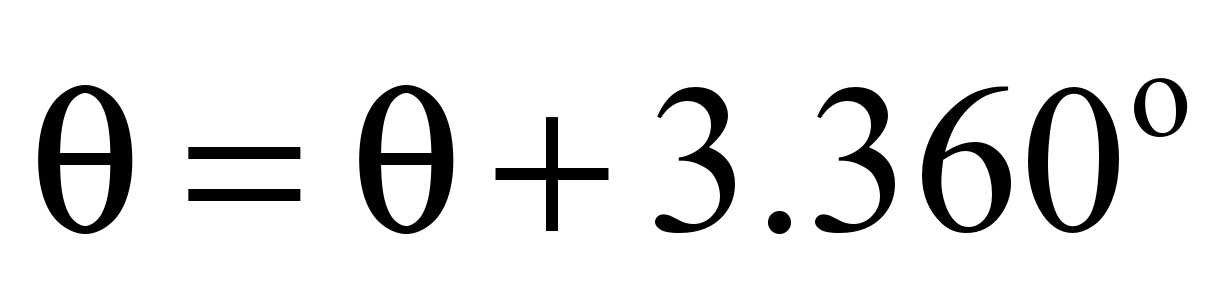mod(360)

.

.

.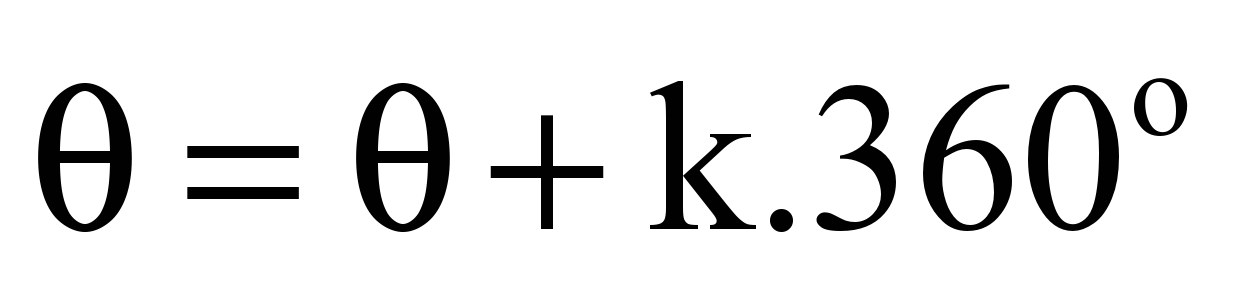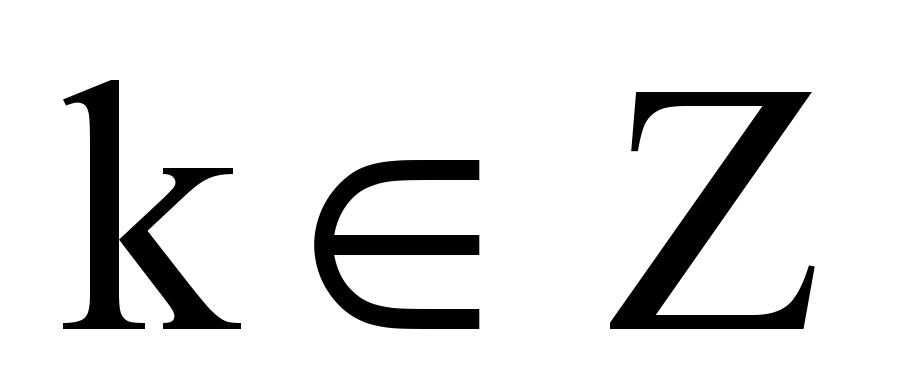mod(360)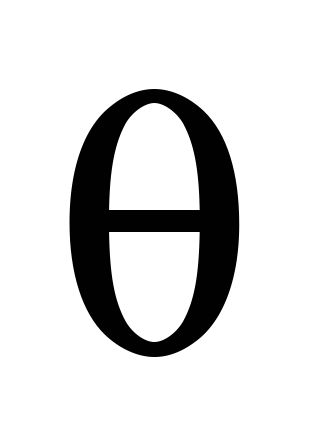is primary directed angle.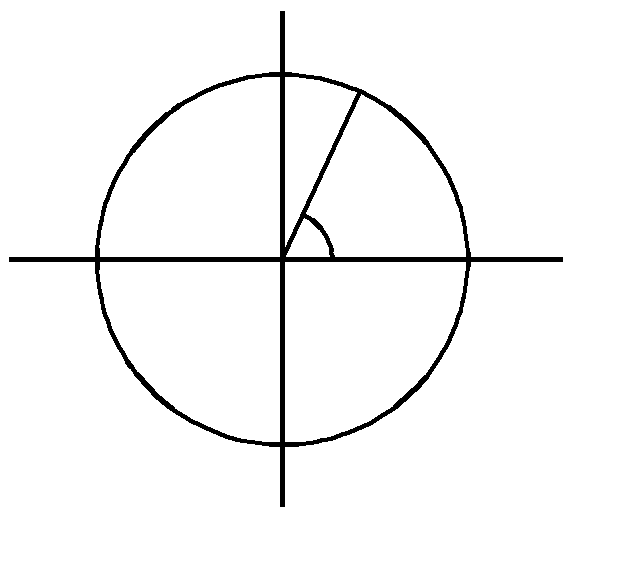Предмет: Математика

Категория: Планирование

Целевая аудитория: 10 класс

Скачать
Introduction to Trigonometry

Автор: Турапбеков Азат Алпысбаевич

Дата: 17.01.2016

Номер свидетельства: 278106

1580 руб.
2260 руб.
1680 руб.
2400 руб.
1860 руб.
2660 руб.
1750 руб.
2500 руб.
1200 руб.
4000 руб.
1200 руб.
4000 руб.
1200 руб.
4000 руб.
900 руб.
3000 руб.
ПОЛУЧИТЕ СВИДЕТЕЛЬСТВО МГНОВЕННО

* Свидетельство о публикации выдается БЕСПЛАТНО, СРАЗУ же после добавления Вами Вашей работы на сайт

Удобный поиск материалов для учителей

Ваш личный кабинет
Проверка свидетельства